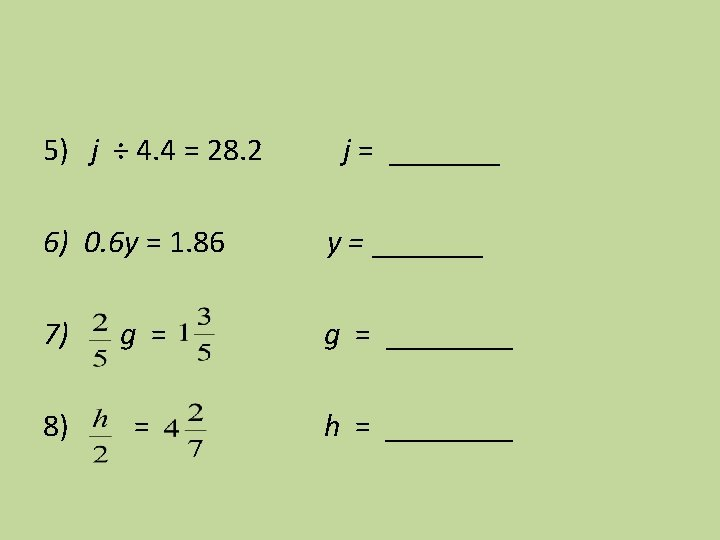# One Step Equations Multiplication and Division What is

• Slides: 15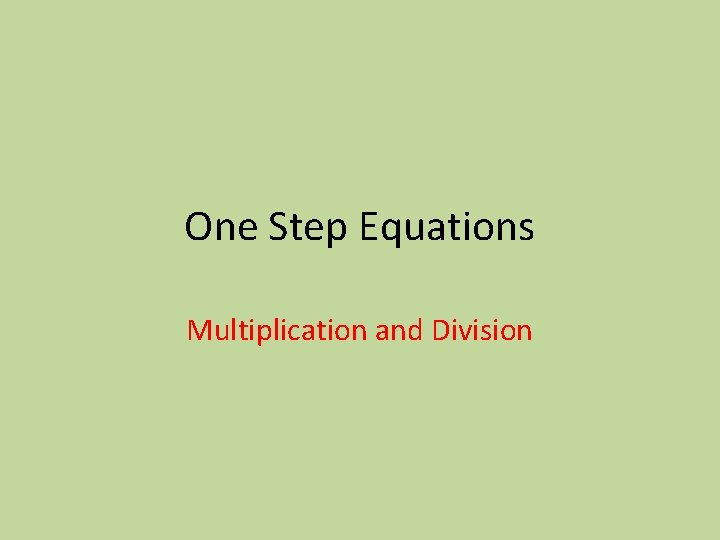One Step Equations Multiplication and DivisionWhat is an equation? • An equation is a mathematical sentence that contains an equal sign ( = ) 5 n = 25 5 n 25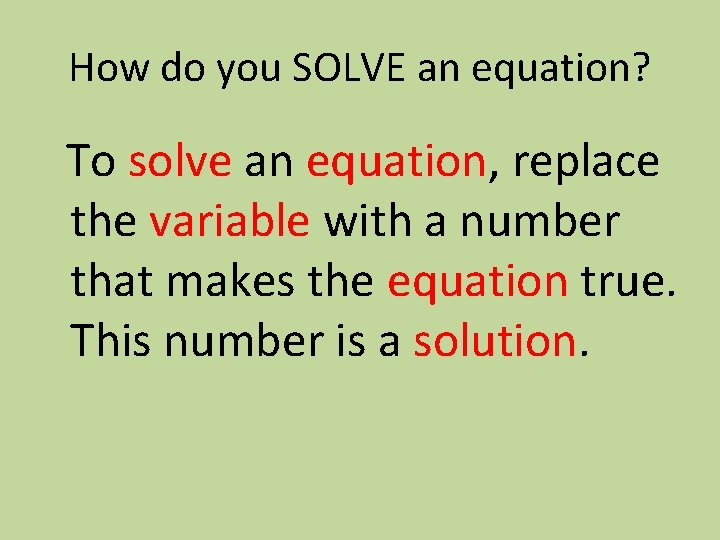How do you SOLVE an equation? To solve an equation, replace the variable with a number that makes the equation true. This number is a solution.Using Substitution to determine the solution. Is 12 the solution to the equation 3 x = 36 3(12) = 36 Substitute x with 12 36 ≠ or = 36 The equation is _______. 12 is or is not the solution to 3 x = 36State whether the given number is a solution to the equation. (yes or no) 1) y ÷ 6 = 4 y = 24 ____ 2) 20 = 5 p p=3 ____ 3) 45 = k 5 4) 8 j = 56 k=9 ____ j=7 ____Solving Equations How do you solve an equation? How do we determine the value of the variable? **Algebra Tiles** **inverse Operation**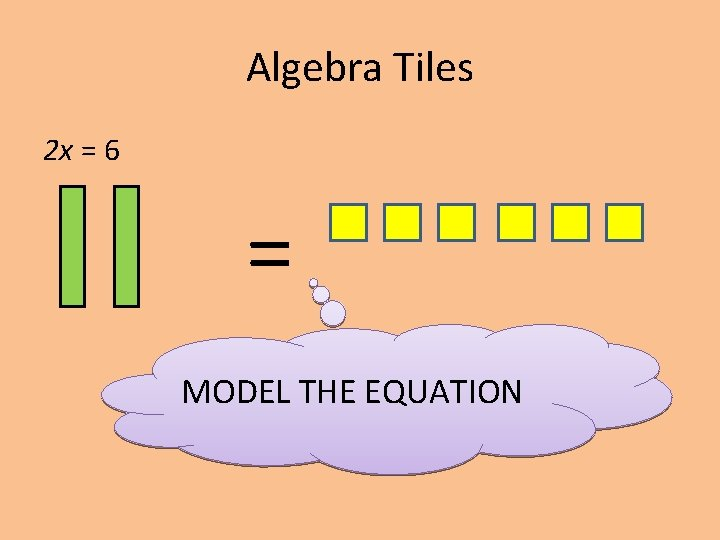Algebra Tiles 2 x = 6 = MODEL THE EQUATIONAlgebra Tiles 2 x = 6 = = Isolate the variables: EACH Variable has to have the same number of tiles!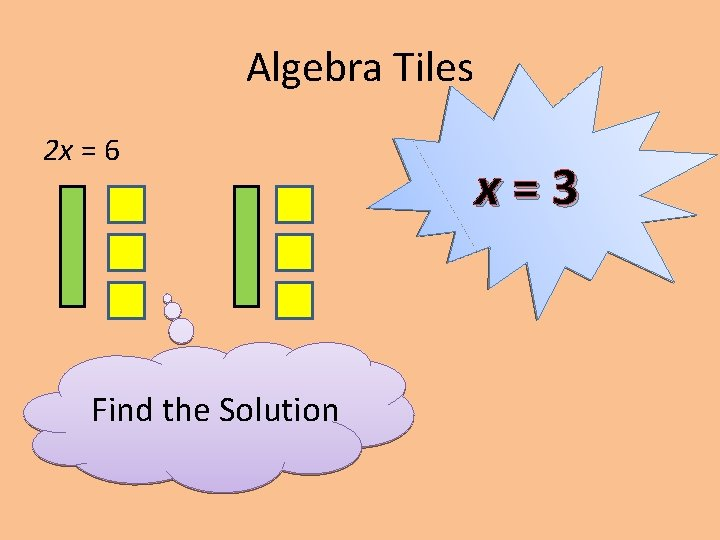Algebra Tiles 2 x = 6 Find the Solution x=3Inverse Operation What is the inverse operation of multiplication? 2 x = 6 2 2 x = 3 Remember an equation is a balance. If you do something to one side you MUST do it to the other.Solve the equation: 4 x = 8 ALGEBRA TILES MODEL: = SOLUTION = X = ______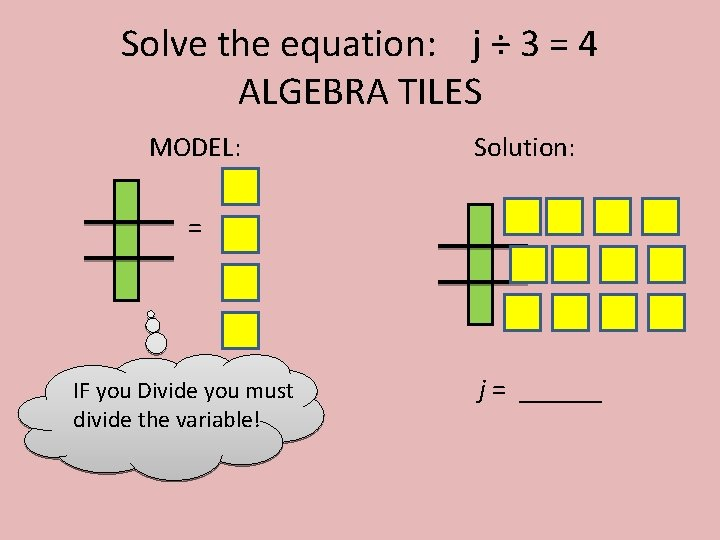Solve the equation: j ÷ 3 = 4 ALGEBRA TILES MODEL: Solution: = IF you Divide you must divide the variable! j = ______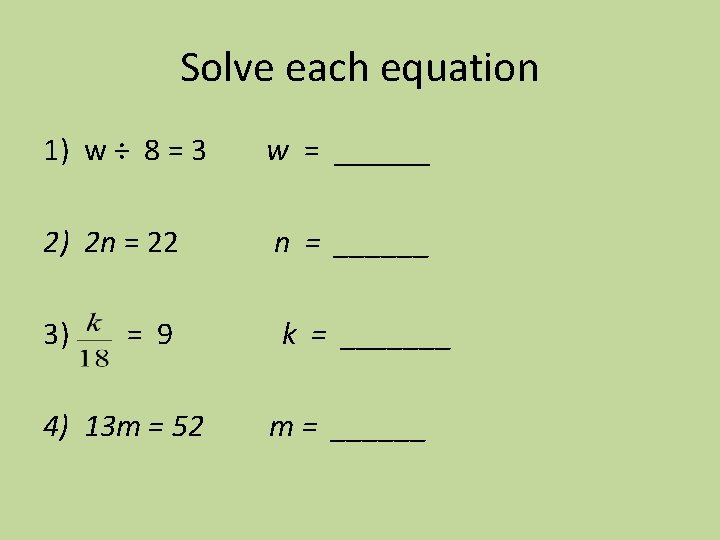Solve each equation 1) w ÷ 8 = 3 w = ______ 2) 2 n = 22 n = ______ 3) k = _______ = 9 4) 13 m = 52 m = ______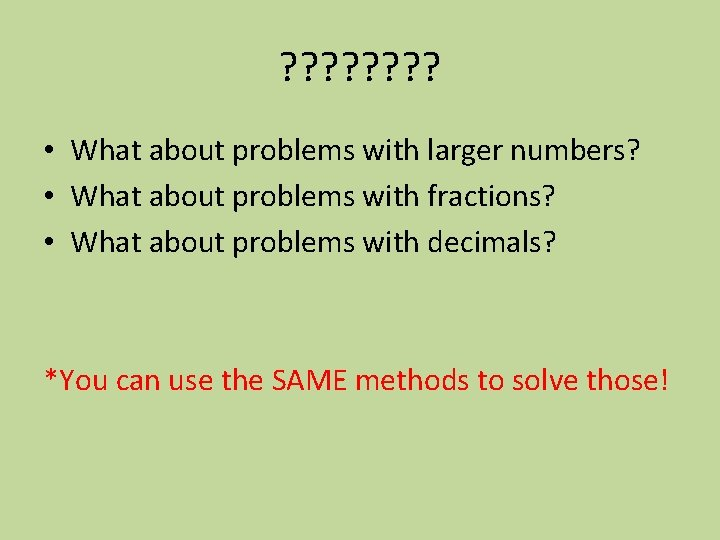? ? ? ? • What about problems with larger numbers? • What about problems with fractions? • What about problems with decimals? *You can use the SAME methods to solve those!